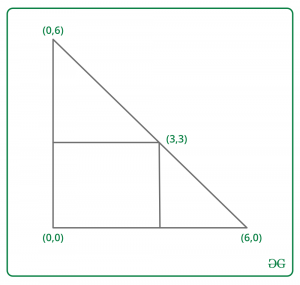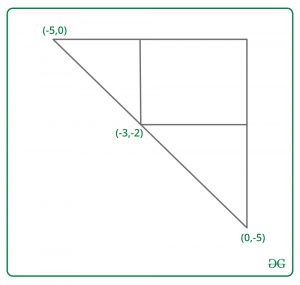# Find two vertices of an isosceles triangle in which there is rectangle with opposite corners (0, 0) and (X, Y)

Given two integers X and Y. The task is to find two vertices of an isosceles triangle ABC(right-angled at B) which has one vertex at a point B(0, 0). And there is a rectangle with opposite sides (0, 0) and (X, Y). All the points of this rectangle are located inside or on the border of the triangle. Print 4 integers x1, y1, x2, y2, where A(x1, y1) and B(x2, y2).

Examples:

Input : X = 3, Y = 3
Output : 6 0 0 6Input : X = -3, y = -2
Output : -5 0 0 -5## Recommended: Please try your approach on {IDE} first, before moving on to the solution.

Approach :
Let Val = |x| + |y|. Then the first point is (Val * sign(x), 0) and the second point is (0, Val * sign(y)).
Let’s see how it works for x > 0 and y > 0. Other cases can be proved in a similar way.
We need to show, that (x, y) belongs to our triangle(including its borders). In fact (x, y) belongs to segment, connecting (x + y, 0) with (0, x + y). The line through (x + y, 0) and (0, x + y) is Y = – X + x + y. Using coordinates (x, y) in this equation proves our answer.

Below is the implementation of the above approach:

## C++

 `// C++ program to find two vertices of an ` `// isosceles triangle in which there is ` `// rectangle with opposite side (0, 0) and (x, y) ` `#include ` `using` `namespace` `std; ` ` `  `// Function to find two vertices of an ` `// isosceles triangle in which there is ` `// rectangle with opposite side (0, 0) and (x, y) ` `int` `Vertices(``int` `x, ``int` `y) ` `{ ` `    ``// Required value; ` `    ``int` `val = ``abs``(x) + ``abs``(y); ` ` `  `    ``// print x1 and y1 ` `    ``cout << val * (x < 0 ? -1 : 1) << ``" 0 "``; ` ` `  `    ``// print x2 and y3 ` `    ``cout << ``"0 "` `<< val * (y < 0 ? -1 : 1); ` `} ` ` `  `// Driver code ` `int` `main() ` `{ ` `    ``int` `x = 3, y = 3; ` ` `  `    ``// Function call ` `    ``Vertices(x, y); ` ` `  `    ``return` `0; ` `} `

## Java

 `// Java program to find two vertices of an ` `// isosceles triangle in which there is ` `// rectangle with opposite side (0, 0) and (x, y) ` `class` `GFG ` `{ ` ` `  `// Function to find two vertices of an ` `// isosceles triangle in which there is ` `// rectangle with opposite side (0, 0) and (x, y) ` `static` `void` `Vertices(``int` `x, ``int` `y) ` `{ ` `    ``// Required value; ` `    ``int` `val = Math.abs(x) + Math.abs(y); ` ` `  `    ``// print x1 and y1 ` `    ``System.out.print(val * (x < ``0` `? -``1` `: ``1``) + ``" 0 "``); ` ` `  `    ``// print x2 and y3 ` `    ``System.out.print(``"0 "` `+ val * (y < ``0` `? -``1` `: ``1``)); ` `} ` ` `  `// Driver code ` `public` `static` `void` `main(String[] args) ` `{ ` `    ``int` `x = ``3``, y = ``3``; ` ` `  `    ``// Function call ` `    ``Vertices(x, y); ` `} ` `} ` ` `  `// This code is contributed by Rajput-Ji `

## Python3

 `# Python3 program to find two vertices of an  ` `# isosceles triangle in which there is  ` `# rectangle with opposite side (0, 0) and (x, y)  ` ` `  `# Function to find two vertices of an  ` `# isosceles triangle in which there is  ` `# rectangle with opposite side (0, 0) and (x, y)  ` `def` `Vertices(x, y) :  ` ` `  `    ``# Required value;  ` `    ``val ``=` `abs``(x) ``+` `abs``(y);  ` ` `  `    ``# print x1 and y1  ` `    ``if` `x < ``0` `: ` `        ``x ``=` `-``1` `    ``else` `: ` `        ``x ``=` `1` `         `  `    ``print``(val ``*` `x,``"0"``,end ``=` `" "``);  ` ` `  `    ``# print x2 and y3  ` `    ``if` `y < ``0` `: ` `        ``y ``=` `-``1` `    ``else` `: ` `        ``y ``=` `1` `     `  `    ``print``(``"0"``,val ``*` `y);  ` ` `  ` `  `# Driver code  ` `if` `__name__ ``=``=` `"__main__"` `: ` ` `  `    ``x ``=` `3``; y ``=` `3``;  ` ` `  `    ``# Function call  ` `    ``Vertices(x, y);  ` ` `  `# This code is contributed by AnkitRai01 `

## C#

 `// C# program to find two vertices of an ` `// isosceles triangle in which there is ` `// rectangle with opposite side (0, 0) and (x, y) ` `using` `System; ` `     `  `class` `GFG ` `{ ` ` `  `// Function to find two vertices of an ` `// isosceles triangle in which there is ` `// rectangle with opposite side (0, 0) and (x, y) ` `static` `void` `Vertices(``int` `x, ``int` `y) ` `{ ` `    ``// Required value; ` `    ``int` `val = Math.Abs(x) + Math.Abs(y); ` ` `  `    ``// print x1 and y1 ` `    ``Console.Write(val * (x < 0 ? -1 : 1) + ``" 0 "``); ` ` `  `    ``// print x2 and y3 ` `    ``Console.Write(``"0 "` `+ val * (y < 0 ? -1 : 1)); ` `} ` ` `  `// Driver code ` `public` `static` `void` `Main(String[] args) ` `{ ` `    ``int` `x = 3, y = 3; ` ` `  `    ``// Function call ` `    ``Vertices(x, y); ` `} ` `} ` ` `  `// This code is contributed by Rajput-Ji `

Output:

```6 0 0 6
```

Time Complexity : O(1)My Personal Notes arrow_drop_uppawanasipugmailcom

If you like GeeksforGeeks and would like to contribute, you can also write an article using contribute.geeksforgeeks.org or mail your article to contribute@geeksforgeeks.org. See your article appearing on the GeeksforGeeks main page and help other Geeks.

Please Improve this article if you find anything incorrect by clicking on the "Improve Article" button below.

Improved By : AnkitRai01, Rajput-Ji